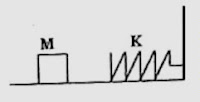## Tuesday, September 04, 2007

### Multiple Choice Questions on Work and Energy

Here is a simple question which is meant for gauging your understanding of the work-energy principle. This MCQ appeared in AIEEE 2005 question paper:The block of mass M moving on the frictionless horizontal surface collides with the spring of spring constant K and compresses it by length L. The maximum momentum of the block after collision is

(a) ML2/K (b) zero (c) KL2/2M (d) √(MK).L

If the maximum momentum of the block after the collision is ‘p’ the maximum kinetic energy is p2/2M. This must be equal to the maximum potential energy of the spring so that we have

p2/2M = ½ KL2, from which p = L√(MK)., given in option (d).

Now consider the following MCQ which appeared in Kerala Medical Entrance 2006 question paper:

The work done by a force F = –6x3 î newton, in displacing a particle from x = 4m to x = 2m is

(a) 360 J (b) 240 J (c) 240 J (d) – 360 J (e) 408 J

This is a case of variable force (in the X-direction), the point of application of which is moved in the X-direction. The work done is therefore given by

W = ∫F.dx = 4 2 (–6x3)dx = –6[x4/4] with x between limits 4 and –2.

Therefore, W = –(6/4)(16 – 256) = 360 joule, given in option (a).

The following MCQ appeared in Kerala Engineering entrance 2006 question paper:

A running man has the same kinetic energy as that of a boy of half the mass. The man speeds up by 2 ms–1 and the boy changes his speed by ‘x’ ms–1 so that the kinetic energies of the boy and the man are again equal. Then ‘x’ in ms–1 is

(a) 2√2 (b) + 2√2 (c) √2 (d) 2 (e) 1/√2

If the mass of the man is ‘m’, the mass of the boy is m/2. If v1 and v2 are the initial velocities of the man and boy respectively, we have

½ mv12 = ½ (m/2)v22

Therefore, v2 = v1√2.

On changing the speeds, we have

½ m(v1+2)2 = ½ (m/2)(v2+x)2

On substituting for v2 (=v1√2), the above equation simplifies to

(v1+2)2 = ½ (v1√2+x)2 from which x = 2√2 ms–1, given in option (b).